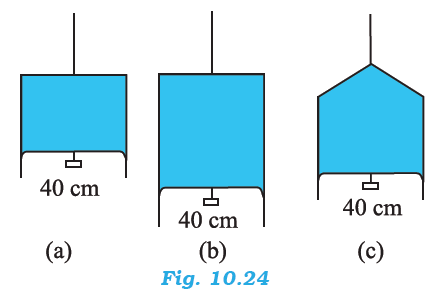## Pages

### Mechanical Properties of Fluids NCERT Solutions Class 11 Physics - Solved Exercise Question 10.18

Question 10.18:
Figure 10.24 (a) shows a thin liquid film supporting a small weight = 4.5 × 10–2 N. What is the weight supported by a film of the same liquid at the same temperature in Fig. (b) and (c)? Explain your answer physically.Solution:
Take case (a):
The length of the liquid film supported by the weight, l = 40 cm = 0.4 cm
The weight supported by the film, W = 4.5 × 10–2 N
A liquid film has two free surfaces.
∴ Surface tension = W / 2l
= 4.5 X 10-2 / (2 X 0.4)  = 5.625 X 10-2 N/m
In all the three figures, the liquid is the same. Temperature is also the same for each case. Hence, the surface tension in figure (b) and figure (c) is the same as in figure (a), i.e., 5.625 × 10–2 N m–1.
Since the length of the film in all the cases is 40 cm, the weight supported in each case is 4.5 × 10–2 N.